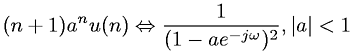Equations > Signal Processing > Fourier Transform Pairs > Discrete-Time Fourier transform of unit step function and exponential

### Discrete-Time Fourier transform of unit step function and exponentialLatex Code:

MathML Code:

 $\left(n+1\right){a}^{n}u\left(n\right)⇔\frac1\left(1-{\mathrm{ae}}^{-j\omega }{\right)}^{2},|a|<1$

MathType 5.0: